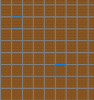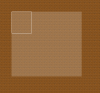# GMS 2[Solved] How can i set a variable collison mask by code?

#### RDM

##### Member
Hey I could really appreciate some help with that problem and please excuse all style problems it's my first post.

Gamemaker Version: 2.1.4.288

How it should work:
I have 2 points that I can move through a grid and when I press a key an object is created at the top left position of an rectangle created by this 2 points.
Then this object/sprite is enlarged to fit the rectangle.
Problem:
The collision mask is not matching the rectangle. I somehow got to change it from it's normal collision mask but i don't know how and it's still too small.

obj_functioncall
Keypress 80(p)
Code:
``````#region //Creation of 2 marker Points
global.p1 = instance_create_layer(mouse_x,mouse_y,"layer_UI",obj_rooms);
global.p2 = instance_create_layer(mouse_x + 50,mouse_y + 50,"layer_UI",obj_rooms);
#endregion``````obj_rooms
keypress 17(STRG)
That part should work but maybe the problem is already here.
Code:
``````#region //setting variables for roomvar p3x = global.p2.x
var p3x = global.p2.x
var p3y = global.p1.y
var p4x = global.p1.x
var p4y = global.p2.y
var px = 0
var py = 0
#region //setting start and end point
var p1_d = point_distance(0,0,global.p1.x,global.p1.y);
var p2_d = point_distance(0,0,global.p2.x,global.p2.y);
var p3_d = point_distance(0,0,p3x,p3y);
var p4_d = point_distance(0,0,p4x,p4y);
var minpd = min(p1_d,p2_d,p3_d,p4_d);
var maxpd = max(p1_d,p2_d,p3_d,p4_d);
if(minpd = p1_d){
global.rx = global.p1.x - 16
global.ry = global.p1.y - 16
}
if(minpd = p2_d){
global.rx = global.p2.x - 16
global.ry = global.p2.y - 16
}
if(minpd = p3_d){
global.rx = p3x - 16
global.ry = p3y - 16
}
if(minpd = p4_d){
global.rx = p4x - 16
global.ry = p4y - 16
}

if(maxpd = p1_d){
px = global.p1.x
py = global.p1.y
}
if(maxpd = p2_d){
px = global.p2.x
py = global.p2.y
}
if(maxpd = p3_d){
px = p3x
py = p3y
}
if(maxpd = p4_d){
px = p4x
py = p4y
}
#endregion
#region //setting width and height always positive
global._width = px - global.rx + 16
if global._width < 0{
global._width = global._width * (-1)
}
global._height = py - global.ry + 16
if global._height < 0{
global._height = global._height * (-1)
}
#endregion
#endregion
#region //insert vars and create room
var new_room = instance_create_layer(global.rx,global.ry,"layer_rooms",obj_rooms_kitchen)
instance_destroy(obj_rooms)
#endregion``````Grid removed to show drawn collision mask

obj_rooms_kitchen
draw
Code:
``````#region //extending sprite to given width and height and making it transparent
draw_sprite_stretched_ext(sprite_index,image_index,rx,ry,_width,_height,c_white,transparent_alpha)
bb_left = 0
bb_top = 0
bb_right = _width
bb_bot = _height
tolerance = 0
draw_rectangle(bbox_left,bbox_top,bbox_right,bbox_bottom,true)

#endregion``````
I tried some variants of setting the collision mask and only this got a change of it.
But I don't know why it always stays at 64x64.

Thanks for any help.

#### jo-thijs

##### Member
Hey I could really appreciate some help with that problem and please excuse all style problems it's my first post.

Gamemaker Version: 2.1.4.288

How it should work:
I have 2 points that I can move through a grid and when I press a key an object is created at the top left position of an rectangle created by this 2 points.
Then this object/sprite is enlarged to fit the rectangle.
Problem:
The collision mask is not matching the rectangle. I somehow got to change it from it's normal collision mask but i don't know how and it's still too small.

obj_functioncall
Keypress 80(p)
Code:
``````#region //Creation of 2 marker Points
global.p1 = instance_create_layer(mouse_x,mouse_y,"layer_UI",obj_rooms);
global.p2 = instance_create_layer(mouse_x + 50,mouse_y + 50,"layer_UI",obj_rooms);
#endregion``````
View attachment 17807

obj_rooms
keypress 17(STRG)
That part should work but maybe the problem is already here.
Code:
``````#region //setting variables for roomvar p3x = global.p2.x
var p3x = global.p2.x
var p3y = global.p1.y
var p4x = global.p1.x
var p4y = global.p2.y
var px = 0
var py = 0
#region //setting start and end point
var p1_d = point_distance(0,0,global.p1.x,global.p1.y);
var p2_d = point_distance(0,0,global.p2.x,global.p2.y);
var p3_d = point_distance(0,0,p3x,p3y);
var p4_d = point_distance(0,0,p4x,p4y);
var minpd = min(p1_d,p2_d,p3_d,p4_d);
var maxpd = max(p1_d,p2_d,p3_d,p4_d);
if(minpd = p1_d){
global.rx = global.p1.x - 16
global.ry = global.p1.y - 16
}
if(minpd = p2_d){
global.rx = global.p2.x - 16
global.ry = global.p2.y - 16
}
if(minpd = p3_d){
global.rx = p3x - 16
global.ry = p3y - 16
}
if(minpd = p4_d){
global.rx = p4x - 16
global.ry = p4y - 16
}

if(maxpd = p1_d){
px = global.p1.x
py = global.p1.y
}
if(maxpd = p2_d){
px = global.p2.x
py = global.p2.y
}
if(maxpd = p3_d){
px = p3x
py = p3y
}
if(maxpd = p4_d){
px = p4x
py = p4y
}
#endregion
#region //setting width and height always positive
global._width = px - global.rx + 16
if global._width < 0{
global._width = global._width * (-1)
}
global._height = py - global.ry + 16
if global._height < 0{
global._height = global._height * (-1)
}
#endregion
#endregion
#region //insert vars and create room
var new_room = instance_create_layer(global.rx,global.ry,"layer_rooms",obj_rooms_kitchen)
instance_destroy(obj_rooms)
#endregion``````
View attachment 17808
Grid removed to show drawn collision mask

obj_rooms_kitchen
draw
Code:
``````#region //extending sprite to given width and height and making it transparent
draw_sprite_stretched_ext(sprite_index,image_index,rx,ry,_width,_height,c_white,transparent_alpha)
bb_left = 0
bb_top = 0
bb_right = _width
bb_bot = _height
tolerance = 0
draw_rectangle(bbox_left,bbox_top,bbox_right,bbox_bottom,true)

#endregion``````
I tried some variants of setting the collision mask and only this got a change of it.
But I don't know why it always stays at 64x64.

Thanks for any help.
Hi and welcome to the GMC!

sprite_collision_mask doesn't return anything, so GameMaker makes it return 0 by default.
When interpreting 0 as a sprite index in GameMaker:Studio, it refers to the first sprite in the resource tree of your project.
Instead, you probably wanted to do this:
Code:
``mask_index = sprite_index;``

A scaled version of itself to match the sprite drawn through draw_sprite_stretched_ext?

#### RDM

##### Member
Thank you
I wanted another way to set the mask as with a sprite.
And yes it should exactly do this but i can't use a sprite because the points are moveable so the scale is always different.(i think i forgot to mention this)

Is there a way to do this?

#### jo-thijs

##### Member
Thank you
I wanted another way to set the mask as with a sprite.
And yes it should exactly do this but i can't use a sprite because the points are moveable so the scale is always different.(i think i forgot to mention this)

Is there a way to do this?
You can set the variables "image_xscale" and "image_yscale" to "_width / sprite_get_width(sprite_index)" and "_height / sprite_get_height(sprite_index)" respectively.
These scales will be applied to the collision mask as well.

#### RDM

##### Member
Thank you very much it works now.
I knew there had to be an easy solution.